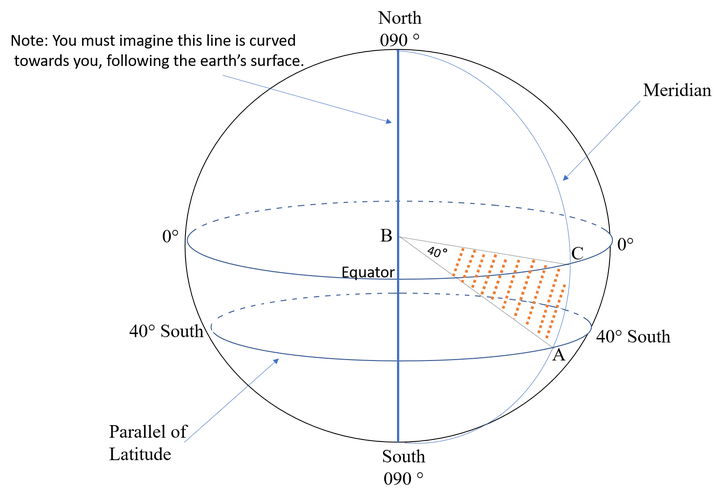# How are Latitude Angles Measured?

Latitude is measured by the angle at the Earth’s centre between the place in question and the equator.This image shows:

• The equator is at 0°
• The latitude of the North Pole is 90°N
• The latitude of the South Pole is 90°S

The latitude of every point between must be either:

• Measured in degrees north from 0° (Equator) to 90°N, or
• Measured in degrees south from 0° (Equator) to 90S°
• This image shows the latitude line at 40° south
• Study the shaded area marked A, B, and C. This shows how the measurement is calculated.
• B is the centre of the Earth
• C is the Equator
• A is the 40° line of latitude

You do not need to worry about finding the angle, as nautical charts provide the degrees of the angle. However, it is important to understand how latitude (and longitude) is found.